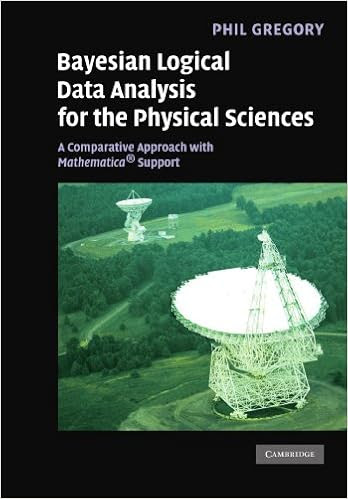## Sunday, September 6, 2015

### Bayesian Logical Data Analysis for the Physical Sciences: A Comparative Approach with Mathematica® Support - 1st Edition by Phil GregoryBayesian inference provides a simple and unified approach to data analysis, allowing experimenters to assign probabilities to competing hypotheses of interest, on the basis of the current state of knowledge. By incorporating relevant prior information, it can sometimes improve model parameter estimates by many orders of magnitude. This book provides a clear exposition of the underlying concepts with many worked examples and problem sets. It also discusses implementation, including an introduction to Markov chain Monte-Carlo integration and linear and nonlinear model fitting. Particularly extensive coverage of spectral analysis (detecting and measuring periodic signals) includes a self-contained introduction to Fourier and discrete Fourier methods. There is a chapter devoted to Bayesian inference with Poisson sampling, and three chapters on frequentist methods help to bridge the gap between the frequentist and Bayesian approaches. Supporting Mathematica® notebooks with solutions to selected problems, additional worked examples, and a Mathematica tutorial are available at www.cambridge.org/9780521150125.